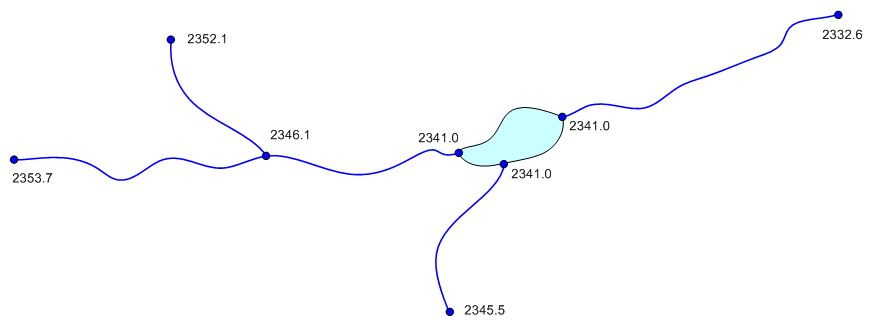# SAMPLE

 Page 1 Page 2 Page 3 Page 4 [Page 5] Page 6

32. (gms) Assume you have been hired to build a steady state MODFLOW model of the groundwater flow conditions in Navajo Valley. A simplified representation of the site is shown below. The boundary of the site to be modelled is shown with a black line. The north and south boundaries correspond to ground water divides and the east and west boundaries correspond to parallel flow boundaries. The blue lines in the middle represent a river. There are four wells and a small lake in the valley.Unzip the archive and load the project into GMS. Then build the model according to the following specifications. Use the Map Module in GMS to build your conceptual model. You may organize the coverages any way you want and complete the model setup in any order as long as you include all of the model features. Use the appropriate packages to represent the features.

a. (1 pts) The four wells all pump at an average rate of 1200 m^3/d.

b. (3 pts) The lake has an average stage of 2341.0 m. The lake bottom sediments are 6.0 m thick and the average vertical K for the sediments is 0.1 m/d.

c. (4 pts) For the river, assign stage values corresponding to the diagram below. Assume that the elevation of the river bottom sediments is 3 m below the stage values.Assume that the river bottom sediments have the following properties.

 Average width: 2.5 m Average vertical thickness: 1.25 m Average vertical K: 0.1 m/day

d. (2 pts) The annual raifall for the area is 2.1 m/yr. Assume that 15% of this value reaches the groundwater table. Use a constant value of recharge for the entire area.

e. (1 pts) Use a constant value K for the aquifer = 1.5 m/d.

f. (1 pts) The aquifer is unconfined and is underlain by bedrock. Assume a constant elevation for the top of bedrock of 2000 m. Use a constant top elevation for your grid of 2400 m.

g. (2 pts) Use a single layer grid with 90 columns (x) and 40 rows (y).